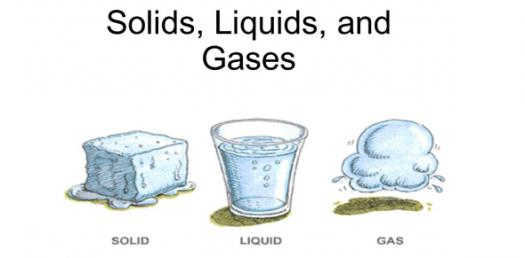# Solids, Liquids, And Gases! Chemistry Trivia Facts Quiz

18 Questions | Attempts: 121SettingsAccording To Science, the matter is anything that has mass and occupies space. Matter exists in generally three states, which are solids, liquids, and gases. In this chemistry trivia facts quiz, you will get to test out how much you know about these three states and the processes they undergo. How about you check it out and see what new facts you might learn.

• 1.
The kinetic theory is an explanation of how particles in matter behave.
• A.

True

• B.

False

• 2.
Not all matter is composed of small particles
• A.

True

• B.

False

• 3.
The particles are  ______________ with each other and the walls of their container.
• A.

Moving

• B.

Colliding

• C.

Jumping

• 4.
Atoms in ________________ are held tightly in place by the attraction between the particles.
• A.

Gases

• B.

Liquids

• C.

Solids

• 5.
_______________means the average kinetic energy of particles in the substance, or how fast the particles are moving.
• A.

Temperature

• B.

Heat

• C.

Kelvin

• 6.
___________________is the pressure of the vapor in a liquid equal to the external pressure on the liquid.
• A.

Melting point

• B.

Boiling point

• C.

Heat of fusion

• 7.
Water freezes at
• A.

0 c

• B.

100 c

• C.

Any temperature

• 8.
A state with no definite shape and definite volume
• A.

Liquid

• B.

Solid

• C.

Gas

• 9.
Gases do not have a fixed volume or shape.
• A.

True

• B.

False

• 10.
Is the amount of energy required for the liquid at its boiling point to become a gas.
• A.

Heat of melting

• B.

Heat of vaporization

• C.

Heat of fusion

• 11.
Is matter consisting of positively and negatively charged particles, found in the sun's core.
• A.

Solid

• B.

Liquid

• C.

Plasma

• 12.
Thermal expansion
• A.

Is a decrease in the size of a substance when the temperature is increased.

• B.

Is an increase in the size of a substance when the temperature is increased.

• C.

Is an increase in the size of a substance when the temperature is decreased.

• 13.
The air in the balloon is heated, causing the distance between the particles in the air to increase.
• A.

Plasma

• B.

Thermal contraction

• C.

Thermal expansion

• 14.
The particles in the liquid in the narrow thermometer tube start to move farther apart as their motion increases.
• A.

Thermal contraction

• B.

Thermal expansion

• C.

Fusion

• 15.
What is the strange behavior of water?
• A.

Expands when heated

• B.

Expands when cooled.

• C.

Contracts when melted

• 16.
These solids lack the highly ordered structure found in crystals, and don't have a definite temperature to melt like volcanic glass.
• A.

Amorphous solids

• B.

Plasma solids

• C.

Crystalline solids

• 17.
Substances start to flow during the melting phase similar to a liquid, but they do not lose their ordered arrangement completely, as most substances do like (LCD) screens.
• A.

Crystalline solids

• B.

Liquid crystals

• C.

Amorphous solids

• 18.
Thermal energy is
• A.

Total of kinetic and potential energy of the particles

• B.

Kinetic energy of particles

• C.

Potential energy of particles

## Related TopicsBack to top
×

Wait!
Here's an interesting quiz for you.